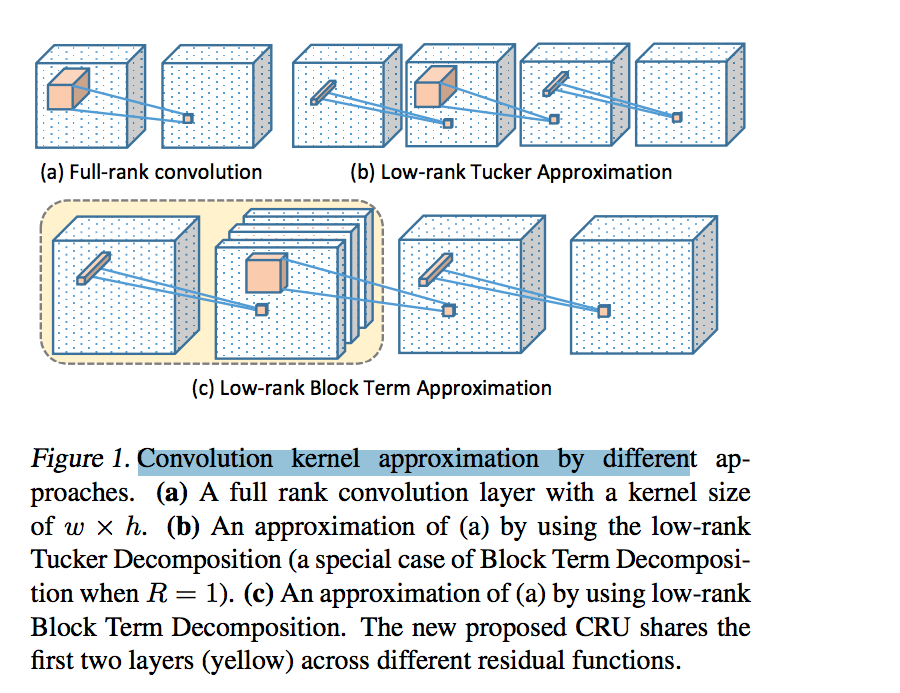## BTD分解概览

2008年Lieven De Lathauwer 等人提出了一种Block term decomposition（BTD），这种张量分解在一些论文中也被称为Block component decomposition (BCD)。我本人认为叫BCD 更合理一些，因为这种张量分解将一个N阶张量分解为R个成员张量的形式，BCD更符合。如下图就是一个$Rank-(L_r, M_r, N_r)$的分解，每一个成员张量是一个$Rank-(L_r, M_r, N_r)$的Tucker分解。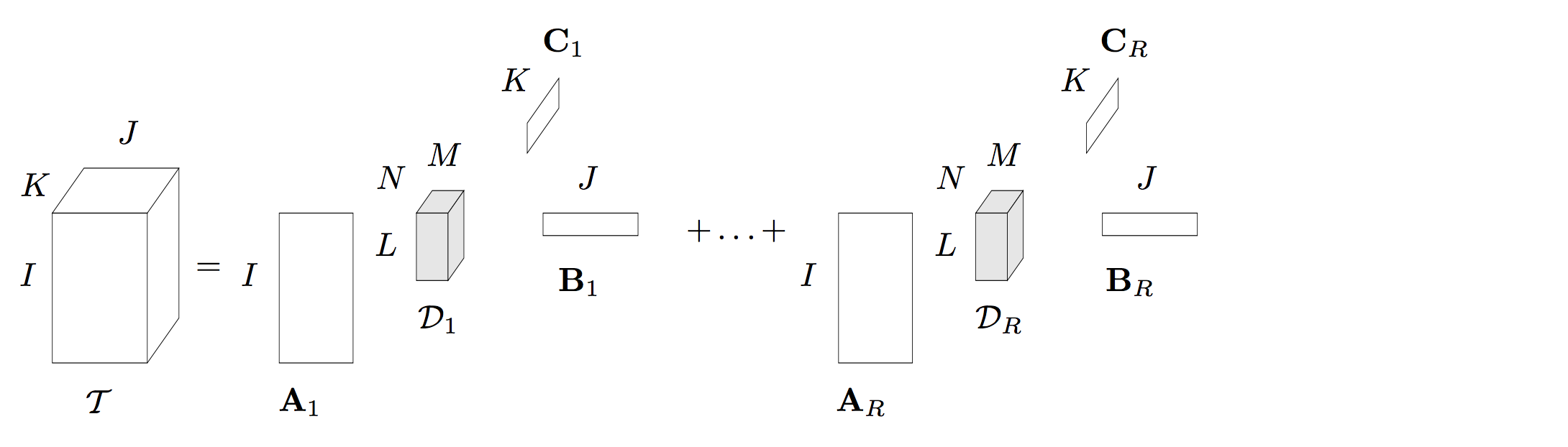\begin{equation} \mathcal{T}=\sum \limits_{r = 1}^{R} \mathcal{D_r}\times_{1} A_r \times_{2} B_r\times_{3}C_r\label{eq:tucker} \end{equation}

## BTD分解的求解

BTD分解现在的求解算法有很多，比如说非线性最小二乘法,交替最小二乘算法（ALS），张量对角算法等,其中交替最小二乘算法形式最为简单，下面我们以一个三阶张量$\mathcal{T} \in ^{I\times J \times K}$的张量为例，这种求解算法进行介绍。

\begin{equation} T_{JK\times I}=(B\otimes C). Blockdiag（\mathcal{D}_{1_{MN\times L}}, \cdots,\mathcal{D}_{R_{MN\times L}}）A^{T} \end{equation}

\begin{equation} T_{KI\times J}=(C\otimes A). Blockdiag（\mathcal{D}_{1_{NL\times M}}, \cdots,\mathcal{D}_{R_{NL\times M}}）B^{T} \end{equation}

\begin{equation} T_{IJ\times K}=(A\otimes B). Blockdiag（\mathcal{D}_{1_{LM\times N}}, \cdots,\mathcal{D}_{R_{LM\times N}}）C^{T} \end{equation}

\begin{equation} A=M^{\dagger}.T_{JK\times I} \end{equation}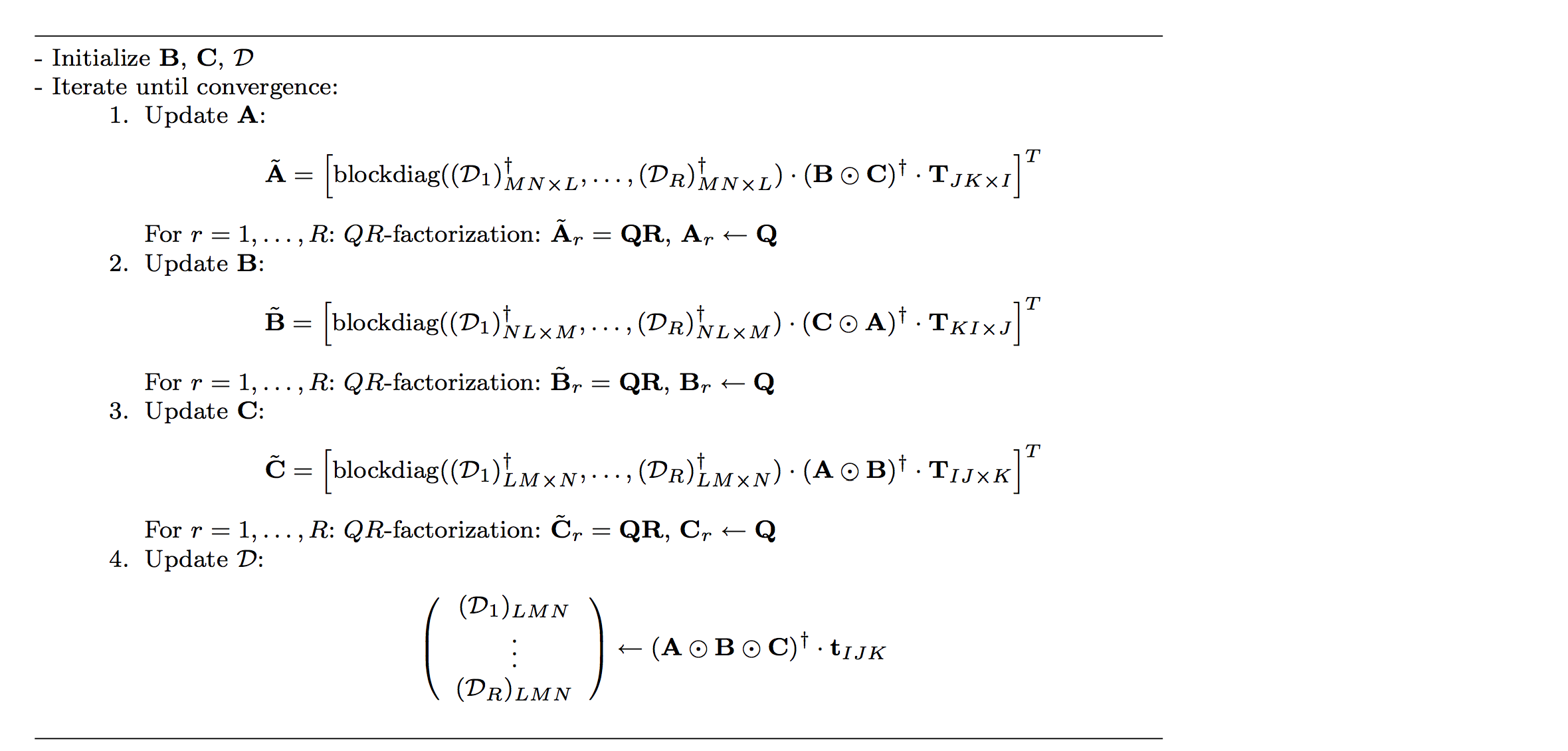## 特殊BTD分解

#### Rank-（Lr,Lr,1）分解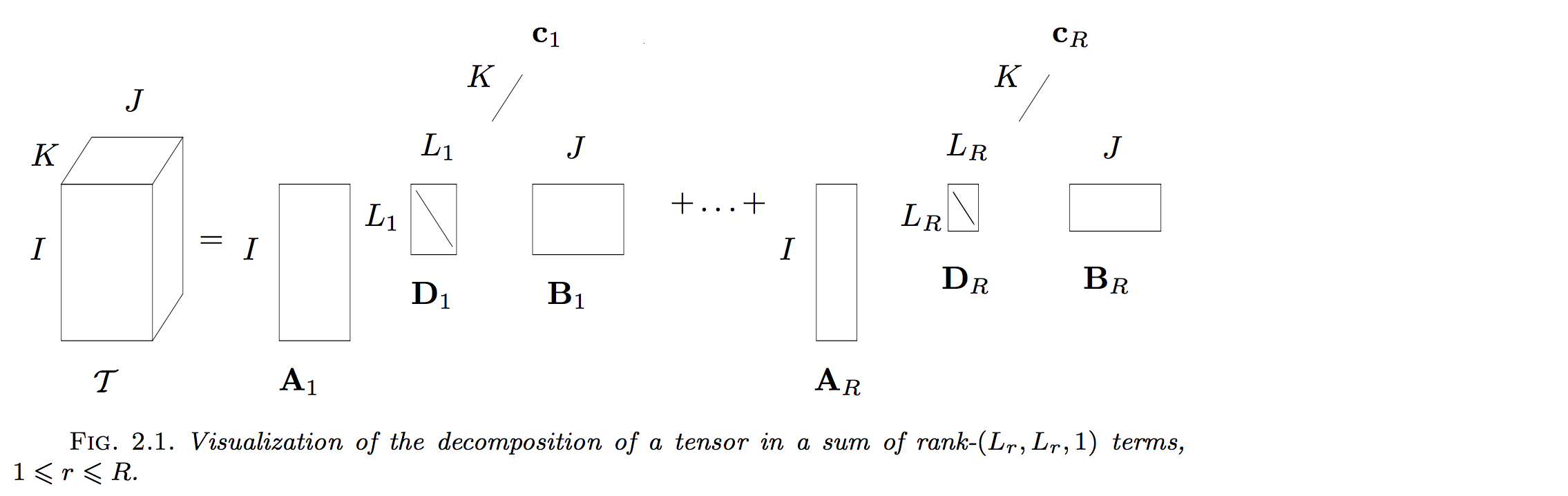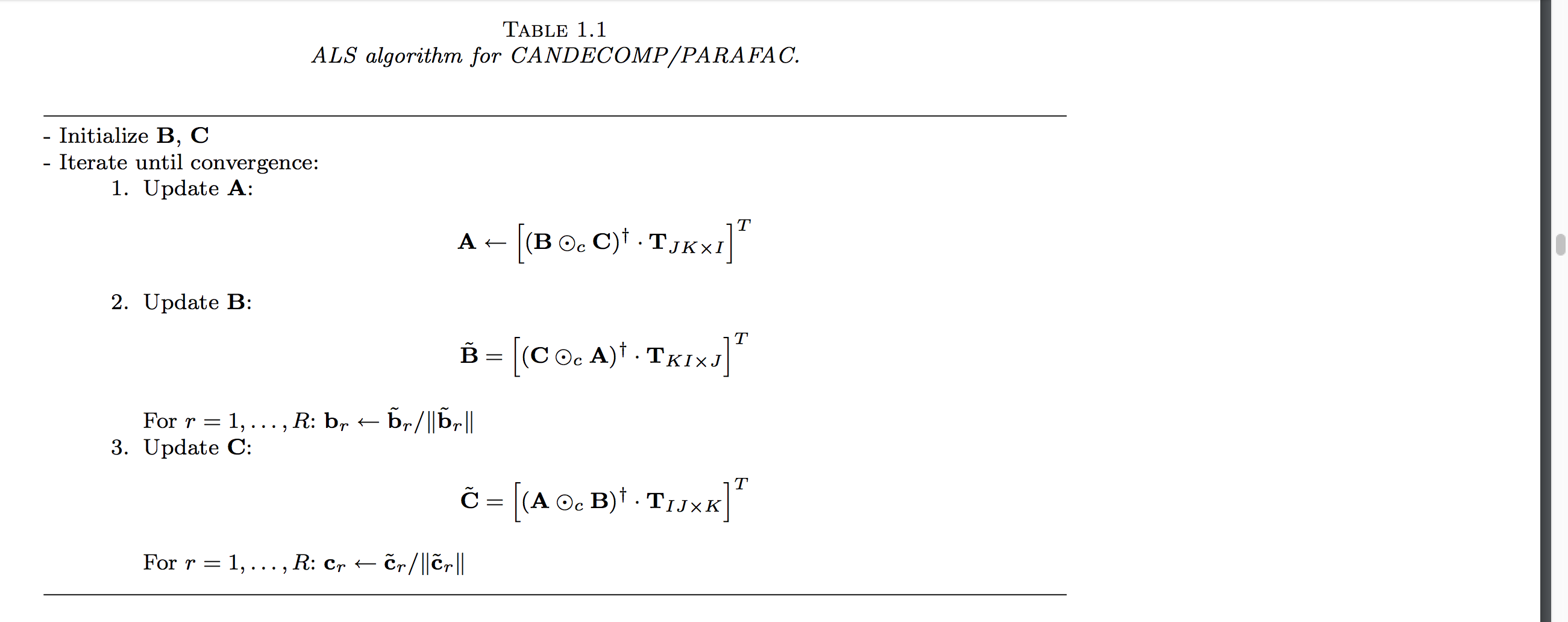#### Rank-（L,L,1）分解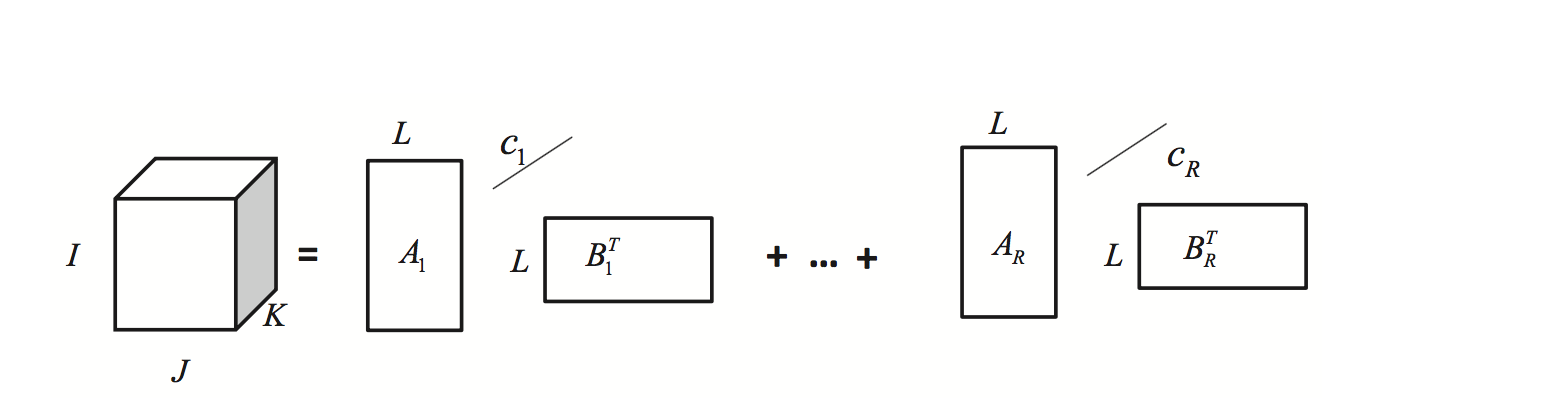## BTD分解的应用

BTD分解的现阶段的主要应用在盲源分离上，尤其是它的蜕化形式$Rank-(L,L,1)$形式。 在$Rank-(L,L,1)$中$c_r$可以看作是一个信号，$A_r\times B_r$可以看作是混合矩阵的低秩表达形式。本人也基于此分解在IEEE TGRS上发表了一篇题为“Matrix-Vector Nonnegative Tensor Factorization for Blind Unmixing of Hyperspectral Imagery”的文章，具体可以看 http://www.cs.zju.edu.cn/people/qianyt/paper/Matrix-Vector%20Nonnegative%20Tensor%20Factorization%20for%20Blind%20Unmixing%20of%20Hyperspectral%20Imagery.pdf。